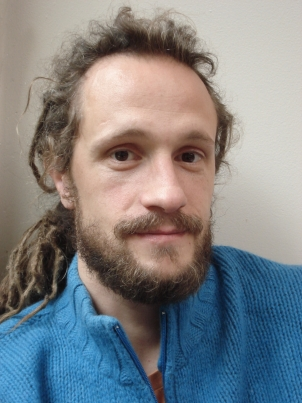STATISTICAL PHYSICS

Outline of the course:
Introduction

From a mechanical to a statistical description of systems of many particles; The notion of equilibrium; Examples of application of statistical physics in modern science and interconnections with other disciplines and everyday life.

Elements of probability theory

Basic notions and definitions; Simple probability distributions (Poisson, Gauss, etc); The central limit theorem.

Postulate of equal a priori probabilities and statistical ensembles
• Microcanonical ensemble (phase space, density of states, Liouville theorem, ergodic hypothesis, temperature, entropy and the connection with thermodynamics)
• Canonical ensemble (thermostat, Boltzmann distribution, partition function, entropy and free energy, equipartition theorem, fluctuations)
• Grand-Canonical ensemble (generalized Boltzmann distribution, chemical potential)
• Applications (ideal gas, Maxwell distribution of velocities, Lennard-Jones model of real gases, van der Waals equation of state, magnetic systems, protein folding)
Quantum statistics
• Bose-Einstein and Fermi-Dirac statistics
• Classical limit and Gibbs paradox
• Applications (black body radiation; Bose-Einstein condensation; Fermi gas; specific heat of molecular gases; phonons; neutron stars; quark-gluon plasma; Big Bang cosmology; electrons in a metal; superconductivity)

Density Matrix; Microscopic definitions of heat and work; Master equation and Langevin equation; Irreversibility as an emergent phenomenon for macroscopic systems; Phase transitions.

Prerequisites:

Mandatory:

• Calculus (integral, differential, multidimensional)
• Newtonian mechanics
• Basics of thermodynamics

Useful (not mandatory):

• Basics of analytical mechanics (Lagrangian and Hamiltonian formulations, phase space)
• Elements of probability theory
• Basics of quantum mechanics
Reference books:

Reif “fundamentals of statistical and thermal physics”
Chandler “introduction to modern statistical mechanics”

The statistical physics course is given by Fabio Pietrucci and Julien Serreau.Fabio Pietrucci worked at SISSA (Trieste) and EPFL (Lausanne) before becoming a "maître de conférences" at Sorbonne University in 2014. His research activity is focused on the development and application of new theoretical and computational approaches to study the transformations of matter. He explores the nucleation of crystals, chemical reactions in solution, and the folding of proteins as well as their interaction with other proteins or drugs. The aim is to establish a unified theoretical framework able to reconstruct transformation mechanisms, free-energy landscapes and kinetic rates for a wide range of different systems.Julien Serreau:
Domains of research: inflationary cosmology and physics of strong interactions
Expertise: quantum field theory (finite temperature, out of equilibrium, in curved spacetime)